# Excel Table Formula Builder

If you want to build a calculator but have already set up the formulas in Excel, you can upload your spreadsheet and select a cell to display results from.

Alternatively, you may have a dataset that you'd like your calculator to reference when returning a result.

## 1. Switch to the Excel style table

Once you've created a new formula, you can click Excel table to navigate to the excel style formula builder.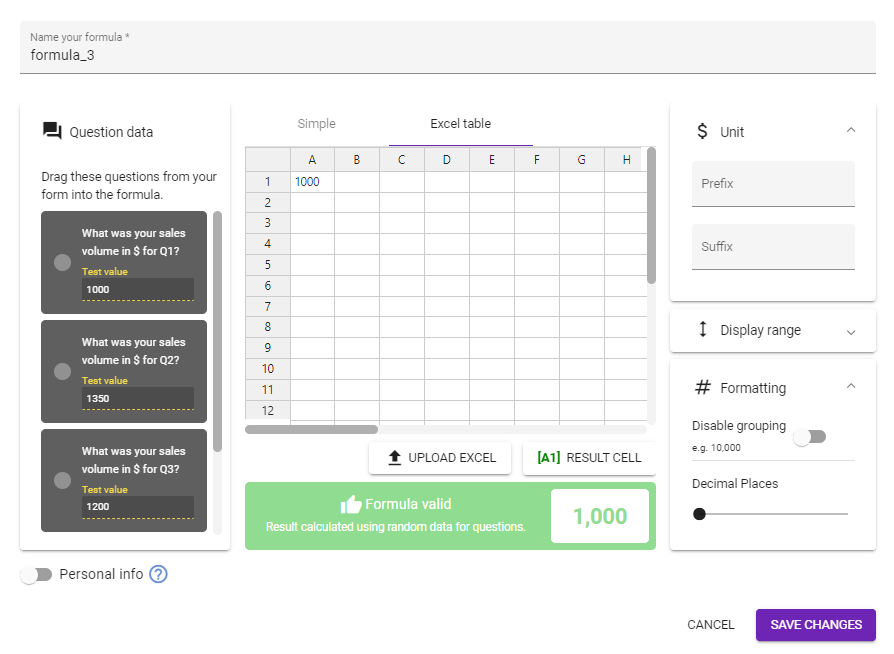Click Upload Excel below the table and select a spreadsheet to import.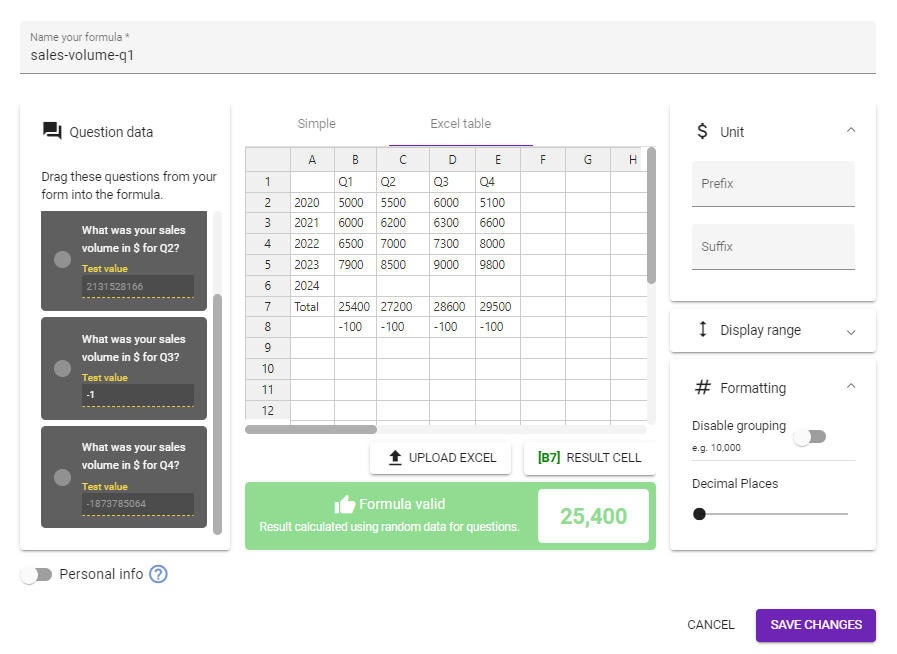Our above example is the total sales volumes for Q1 to Q4 from 2020 to 2023. You'll see a blank row for 2024, which is where we'll map our question cells. But before we do that, let's go over what formulas are already in place so you can see what's happening behind the scenes.

Along row 7, we've got a basic SUM formula in place.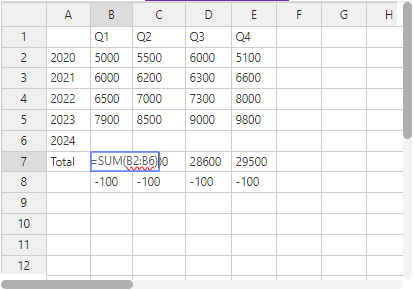We may not use this for this calculation, but it's a nice to have.

Along Row 8, we're calculating the percentage change between sales in Q1 to Q4 in 2023 and 2024.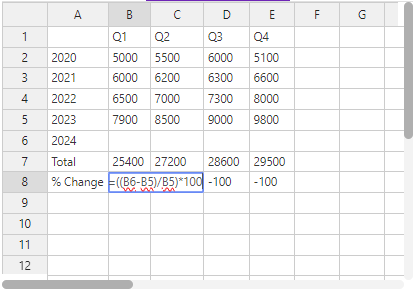This is the value we'll be returning in our calculator. Essentially, we want to display what the percentage increase or decrease was between 2023 Q1 and 2024 Q1.

To do this, we need top map the first question in our calculator into B6, so the value given can be used in our formula.

## 3. Map your questions into cells

To map your questions into cells, all you need to do is drag your question from the left into the desired cell.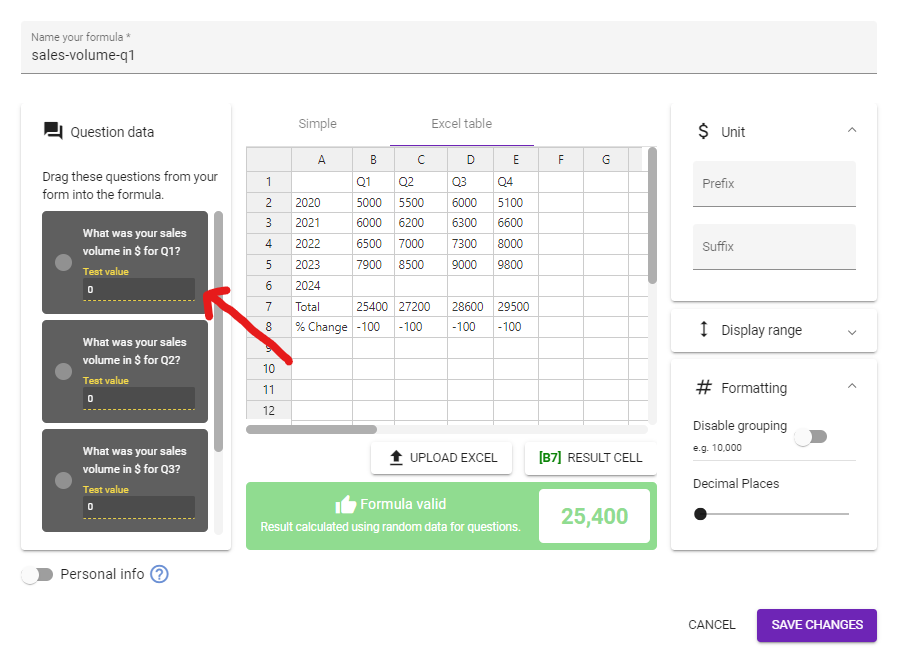After you've dropped the question, a unique question number will be displayed in the cell. This is the formula that dictates the value entered into the cell.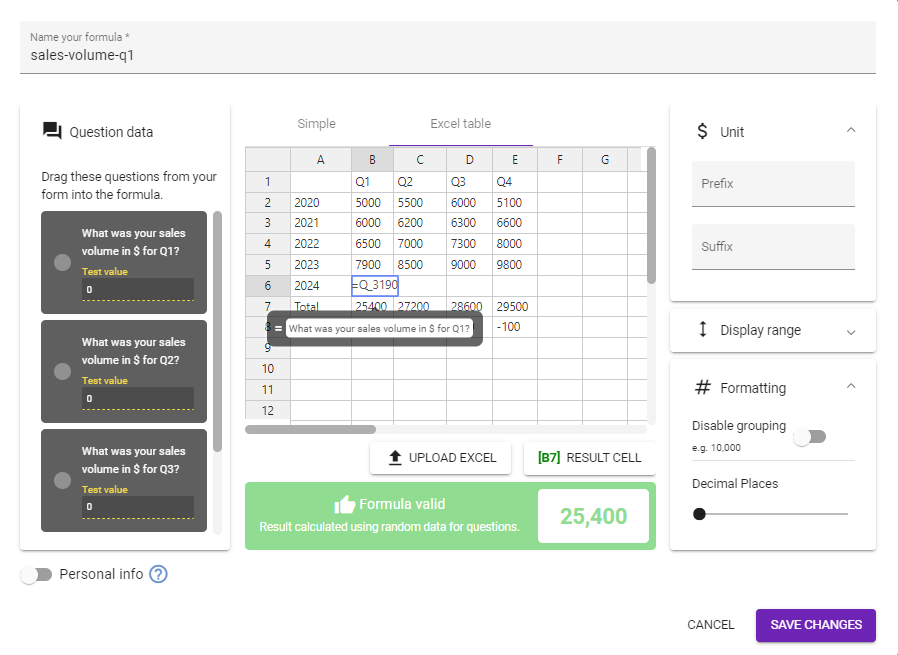Once you click away from the cell, the test value present beneath the question on the left will instead be displayed.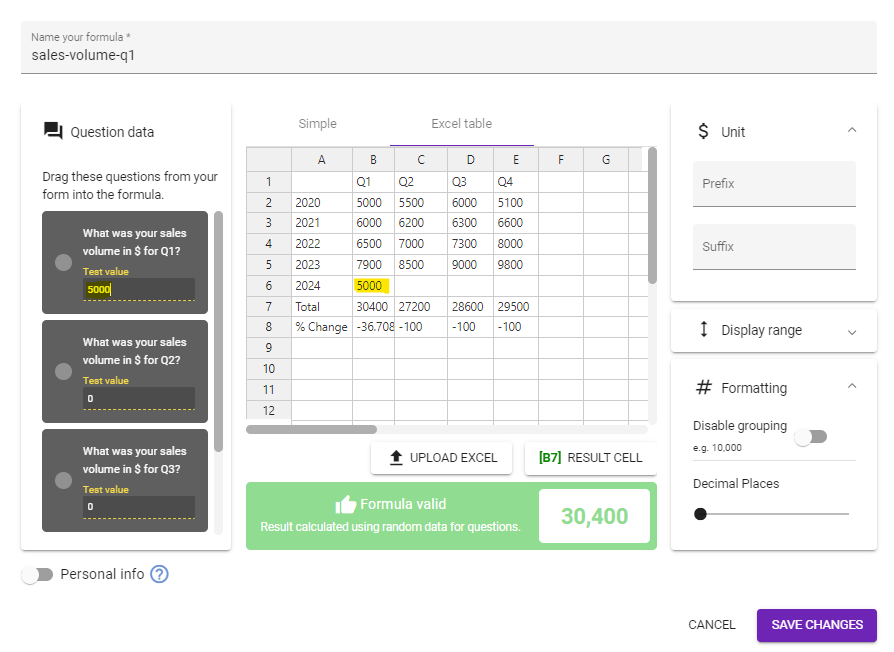## 4. Designate a results cell

Once you've mapped all your question cells, you need to designate a cell that returns a result. In our case, this would be B8, as we want to show the percentage change between B6 and B5.

To choose a results cell, click RESULT CELL beneath the table and enter a cell: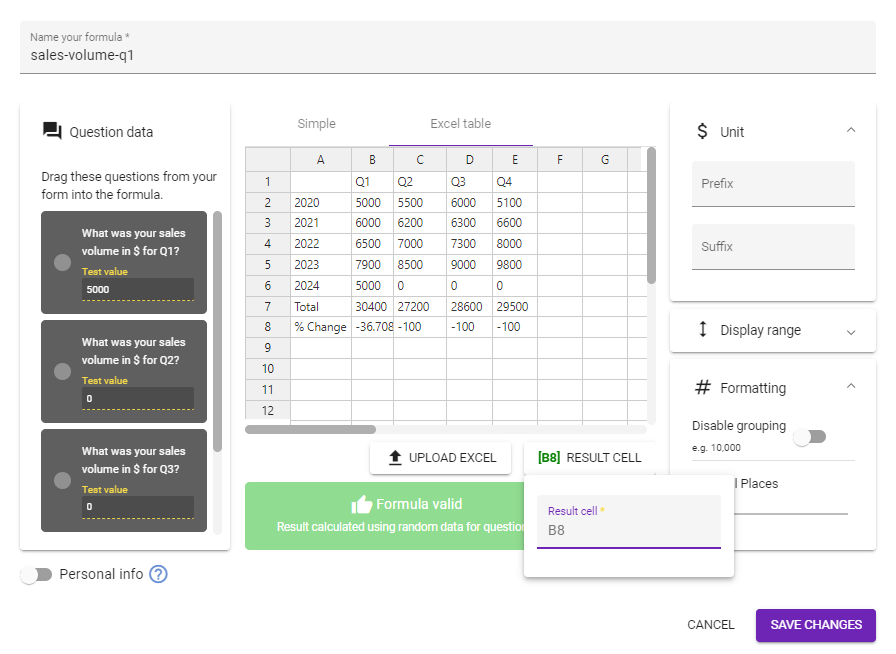Because of the nature of our example, we also want to add a suffix to our respondents know this value is a percentage: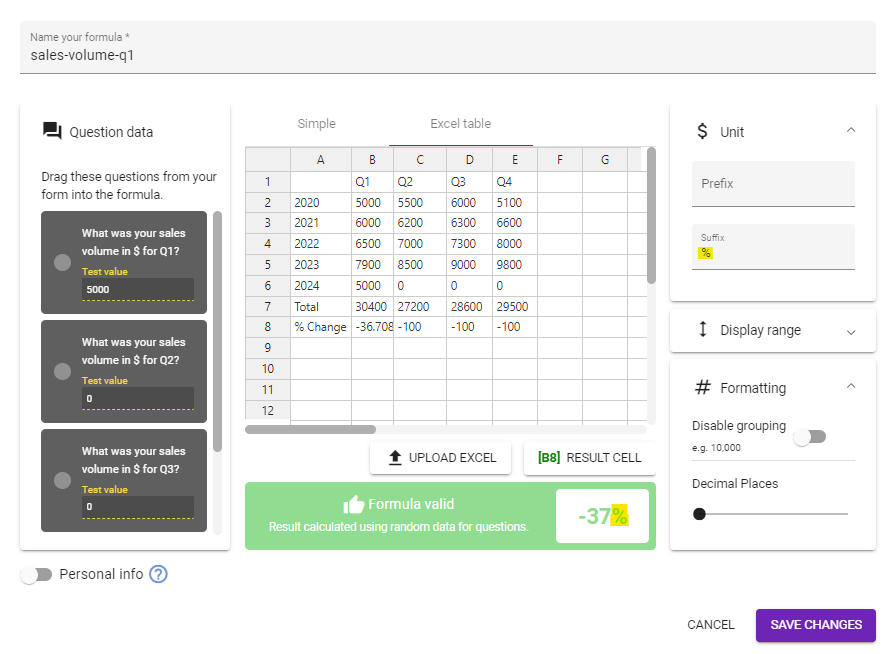Make sure you hit Save Changes to ensure your formula and data is saved.

If you want to show multiple results, you'll need to follow this process for each of the results you want to display.

However, if you're using the same dataset and formulas for each results, we recommend that you duplicate your formula.

Then, all you need to do is access your copied formula and select a different results cell. In the case of the example we've been following, I'd duplicate this and then select C8 to show the results for Q2.